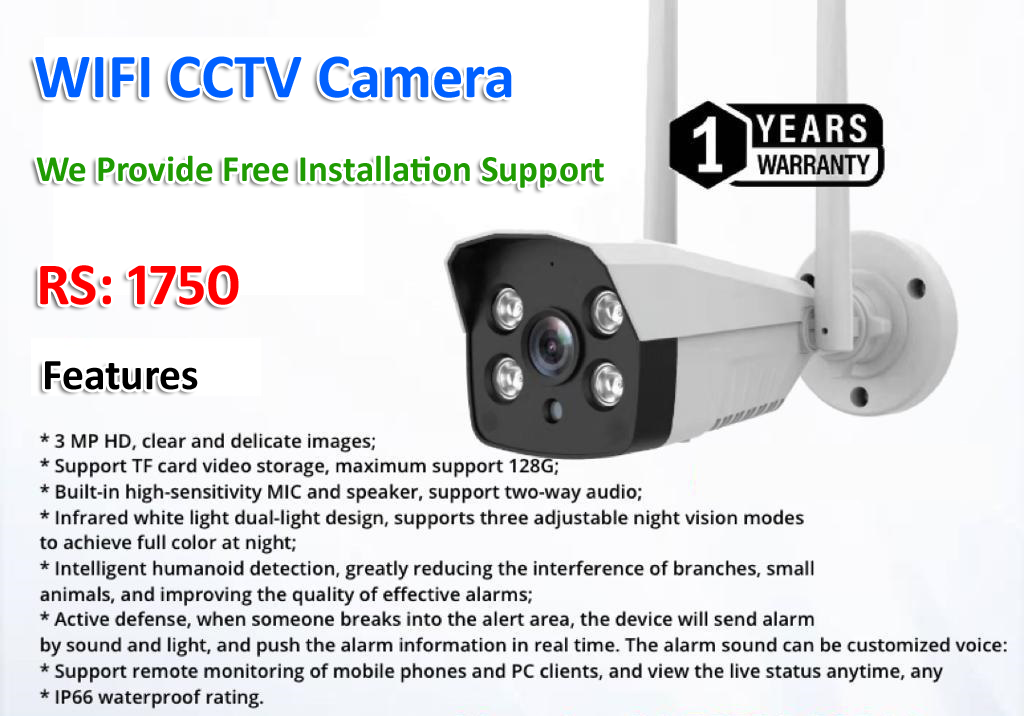# C Program Convert Decimal to Binary

## Convert Decimal to Binary Programs in C

Decimal system is the most widely used number system. But computer only understands binary. Binary, octal and hexadecimal number systems are closely related and we may require to convert decimal into these systems. Decimal system is base 10 (ten symbols, 0-9, are used to represent a number) and similarly, binary is base 2, octal is base 8 and hexadecimal is base 16.

### Concept to Convert Decimal to Binary

To convert integer to binary, start with the integer in question and divide it by 2 keeping notice of the quotient and the remainder. Continue dividing the quotient by 2 until you get a quotient of zero. Then just write out the remainders in the reverse order.

### Example of Convert Decimal to Binary

In below example we convert 13 into Binary

• 13:2=6+1
• 6:2=3+0
• 3:2=1+1
• 1:2=0+1

Now, we simply need to write out the remainder in the reverse order-1101. So, 13 in decimal system is represented as 1101 in binary.

## Decimal to Binary Conversion in C

```#include<stdio.h>
#include<conio.h>

void main()
{
int no,rem,i=0,j;
clrscr();
printf("Please Enter any Decimal Number: ");
scanf("%d",&no);
while(no>0)
{
rem[i]=no%2;
i++;
no=no/2;
}
printf("Binary number is: ");
for(j=i-1;j>=0;j--)
{
printf(" %d",rem[j]);
}
getch();
}
```

## Output

```Please Enter any Decimal Number: 15
Binary number is: 1111
```

WIFI CCTV Camera